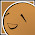:::

# 輸出陣列元素所有不重複組合 print all unique combination of an array 1/04/2011 程式寫作 , JavaScript , Programming/JavaScript 5 Comments Edit Copy Download

#### 程式問題說明

• 輸入：["A", "B", "C"]
• 輸出：[["A"], ["B"], ["C"], ["A", "B"], ["A", "C"], ["B", "C"], ["A", "B", "C"]]

• 輸入：["A", "B", "C", "D"]
• 輸出：[["A"],["B"],["C"],["D"],["A","B"],["A","C"],["A","D"],["B","C"],["B","D"],["C","D"],["A","B","C"],["A","B","D"],["A","C","D"],["B","C","D"],["A","B","C","D"]]

#### 答案程式碼

```function unique_combination_of_array(_array) {
var _combination = [];

var _length = _array.length;

for (var _l = 1; _l < _length; _l++)
{
_combination = parse_combination(_array, _combination, _l);
}
_combination.push(_array);

return _combination;
}

function parse_combination(_array, _output, _length, _length_pos, _length_ang)
{
if (_length_pos == null)
_length_pos = 1;
if (_length_ang == null)
_length_ang = [];

//初始化的部份
if (_length_ang.length < _length_pos)
{
if (_length_pos == 1)
{
_length_ang.push(1);
}
else
{
var _prev_index = _length_ang[(_length_ang.length-1)];
_length_ang.push(_prev_index+1);
}
}

var _index = 0;
if (_length_pos > 1)
{
var _prev_index = _length_ang[(_length_pos-2)];
_index = _prev_index+1;
}

if (_length_ang.length < _length_pos)
{
_length_ang.push(_index);
}

for (var _i = _index; _i < _array.length - (_length - _length_pos); _i++)
{
if (_length_ang.length > _length_pos)
{
//避免受到傳址影響，必須重整
var _temp = [];
for (var _p = 0; _p < _length_pos; _p++)
_temp.push(_length_ang[_p]);
_length_ang = _temp;
}

_length_ang[(_length_pos-1)] = _i;

//如果抵達這個長度的話
if (_length_ang.length == _length)
{
//輸出
var _output_ang = [];
for (var _a in _length_ang)
{
_index = _length_ang[_a];
var _code = _array[_index];
_output_ang.push(_code);
}
_output.push(_output_ang);
}
else    //如果尚未抵達這個長度
{
_output = parse_combination(_array, _output, _length, (_length_pos+1), _length_ang);
}
}

return _output;
}```

#### 總共5 則留言, (我要發問)

1.記得寫這篇的時候，有個朋友問我：「為什麼不要用Java？Java效率不是比較好嗎？」
可是考量到Java還要安裝JDK、編譯、執行結果的時間，用JavaScript直接開啟網頁就能看到結果，不是快上許多嗎？
而且這又不是什麼龐大的程式XD

直到現在，我依然是這樣覺得。
不需要編譯就可以直接運作的JavaScript真是太棒了。

2.你好, 請問這網頁所寫的javascript 程式是否有bugs?
我『新增參數』加了一個 "E" , 再『產生排列組合』....結果仍然是
A
B
C
D
A,B
A,C
A,D
B,C
B,D
C,D
A,B,C
A,B,D
A,C,D
B,C,D
A,B,C,D

煩請賜教, 謝謝!

1.To Haha 1988，

雖然增加參數的部分不太靈光，但是主程式碼的部分倒是沒啥問題
我另外用CodePen寫了一遍，試著玩玩看吧
http://codepen.io/pulipuli/full/oZWdJK/

3.你好，我想請問為甚麼我修改參數，他出來的結果還是只有
A
B
C
D
A,B
A,C
A,D
B,C
B,D
C,D
A,B,C
A,B,D
A,C,D
B,C,D
A,B,C,D
謝謝

1.您好，看程式碼可以找到原因
https://codepen.io/pulipuli/pen/oZWdJK

不過看起來您好像不是來討論程式的，只是想找個工具...？